# Name of All Group members Section At the end of this activity you will be able...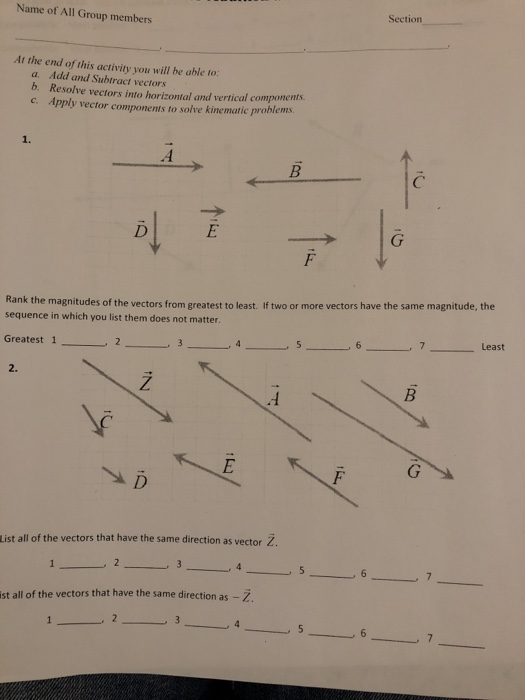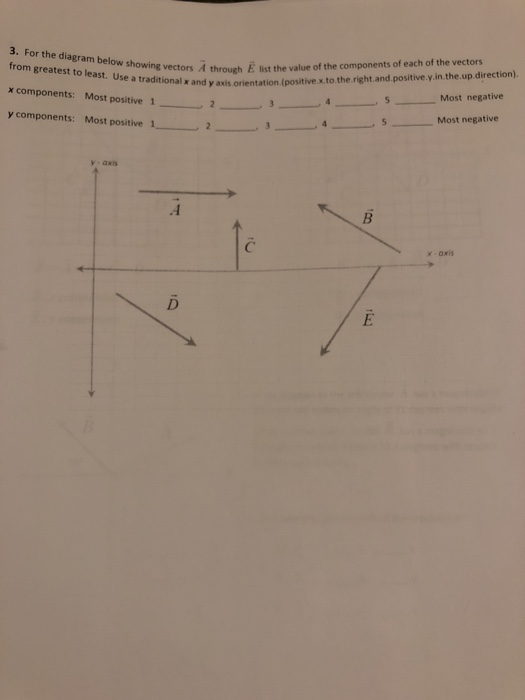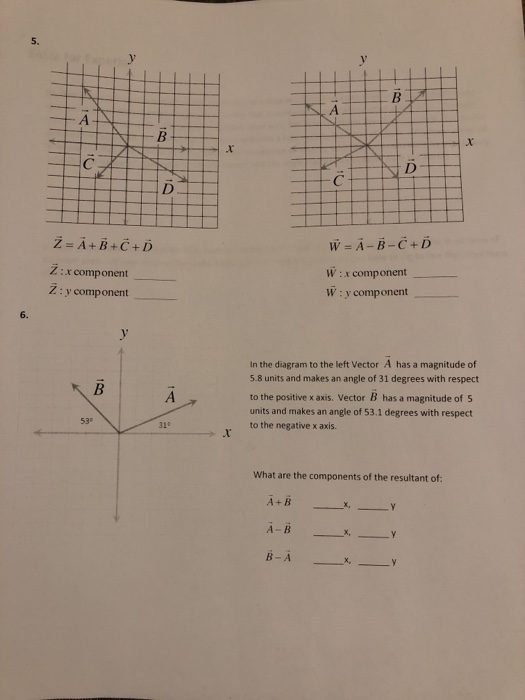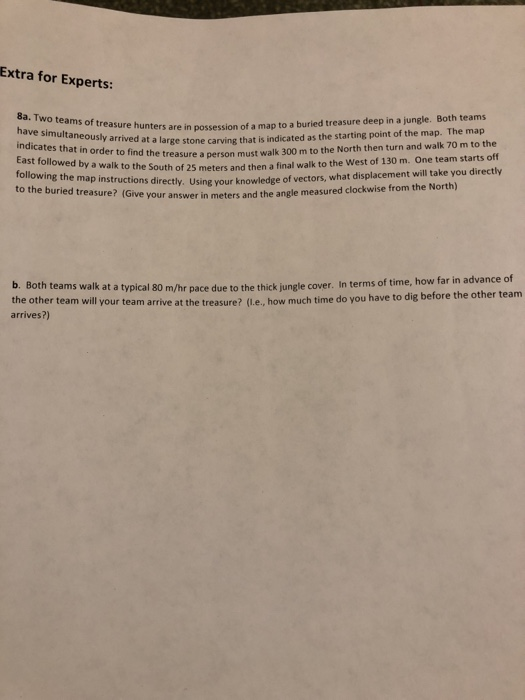Name of All Group members Section At the end of this activity you will be able to: a. Add and Subtract vectors b. Resolve vectors into horizontal and vertical components c. Apply vector components to solve kinematic problems. Rank the magnitudes of the vectors from greatest to least. If two or more vectors have the same magnitude, the sequence in which you list them does not matter. 7Least Greatest 1 2 3 , 4 2. List all of the vectors that have the same direction as vector Z. 2. ist all of the vectors that have the same direction as
3. For the diagram below showing through from greatest to least. Use a traditional x and orientat x components: Most positive 1- 2- y components: Most positive 1 list the value of the components of each of the vectors y axis orientation.(positive.x.to.the.right.and.positive.y.in.the.up.direction). vectors A through 3 , 4 , 5 Most negative Most negative y- axis x-axis
5, W=А-В-С+D W:x component W: y component Z=A+B+C+D Z :x component_ Ž: y component In the diagram to the left Vector A has a magnitude of 5.8 units and makes an angle of 31 degrees with respect to the positive x axis. Vector B has a magnitude of 5 units and makes an angle of 53.1 degrees with respect to the negative x axis. 53 31° What are the components of the resultant of:
Extra for Experts: 8a. Two teams of treasure hunters are in possession of a map to a buried treasure deep in a jungle. Both teams t a large stone carving that is indicated as the starting point of the map. The map indicates that in order to find the treasure a person must walk East followed by a walk to the South of 25 meters and then 300 m to the North then turn and walk 70 m to the a final walk to the West of 130 m. One team starts off s directly. Using your knowledge of vectors, what displacement will take you directly to the buried treasure? (Give you r answer in meters and the angle measured clockwise from the North) b. Both teams walk at a typical 80 m/hr pace due to the thick jungle cover. In terms of time, the other team will your team arrive at the treasure? (ILe., how much time do you have to dig before the ot arrives?)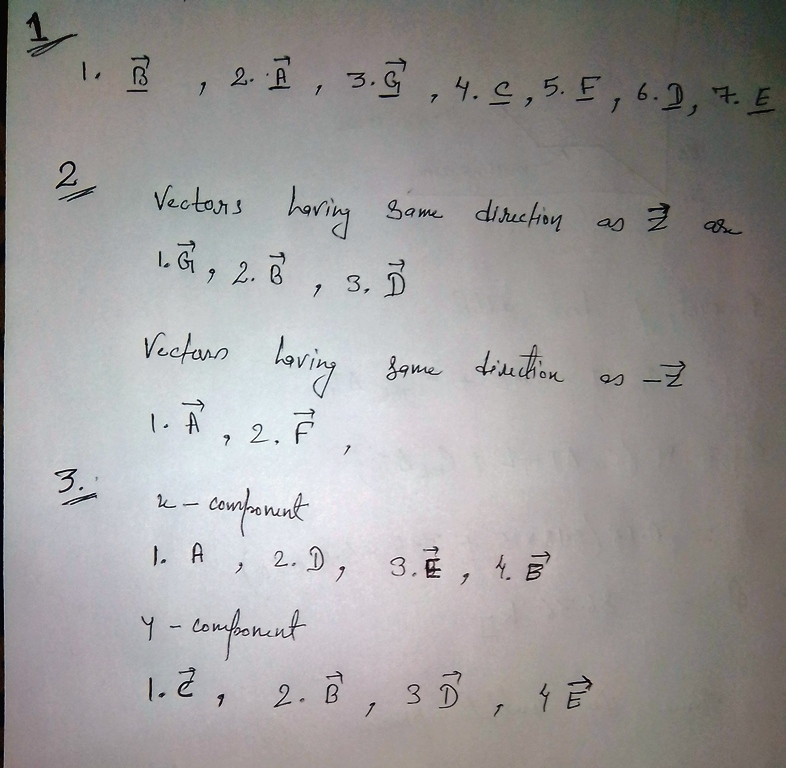#### Earn Coin

Coins can be redeemed for fabulous gifts.

Similar Homework Help Questions
• ### Extra for Experts: 8a. Two teams of treasure hunters are in possession of a map to...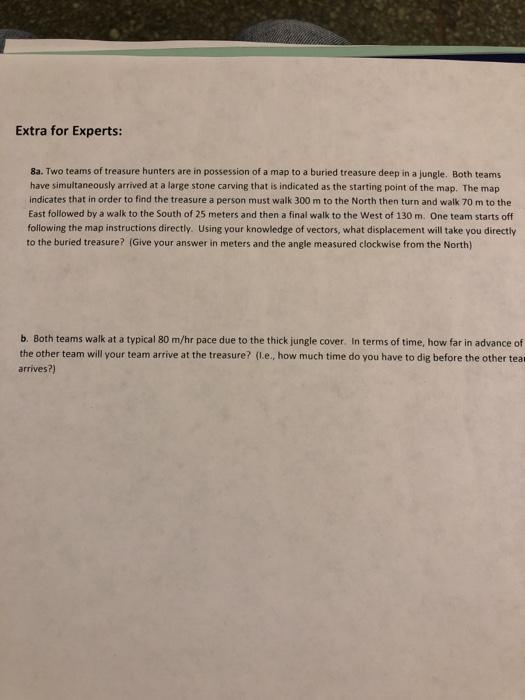Extra for Experts: 8a. Two teams of treasure hunters are in possession of a map to a buried treasure deep in a jungle. Both teams have simultaneously arrived at a large stone carving that is indicated as the starting point of the map. The map indicates that in order to find the treasure a person must walk 300 m to the North then turn and walk 70 m to the East followed by a walk to the South of 25...

• ### 1) What are the x and y components of this vector? x-component: 2) y-component 3)The x...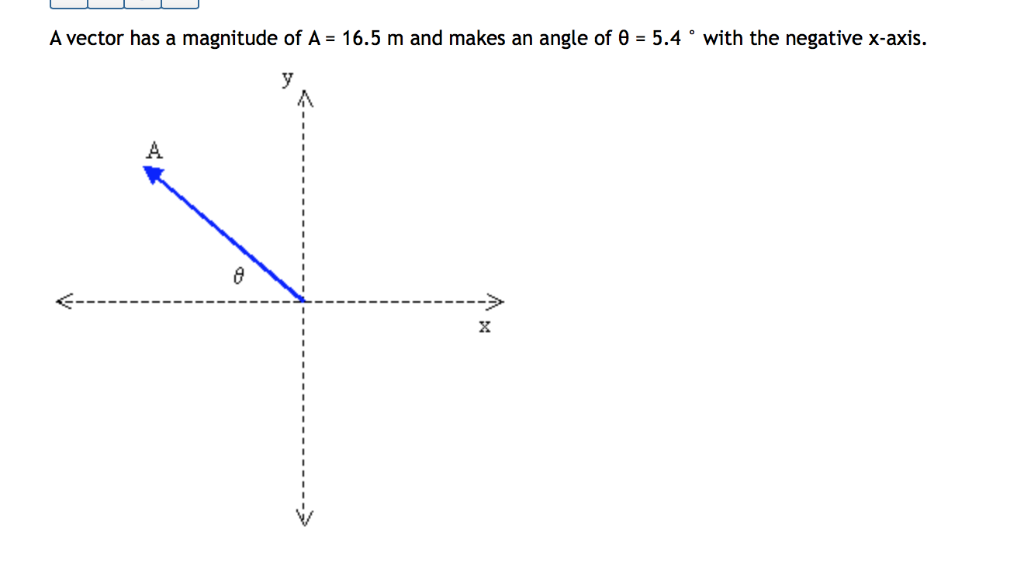1) What are the x and y components of this vector? x-component: 2) y-component 3)The x component of a vector is 12.5 m and the y component of a vector is 25 m. What is the magnitude and direction (with respect to the positive x-axis) of this vector? magnitude: 4)direction: A vector has a magnitude of A = 16.5 m and makes an angle of 0 = 5.4 ° with the negative x-axis. A

• ### Vector A has magnitude 9.0 m and is at an angle of 30 above the negative...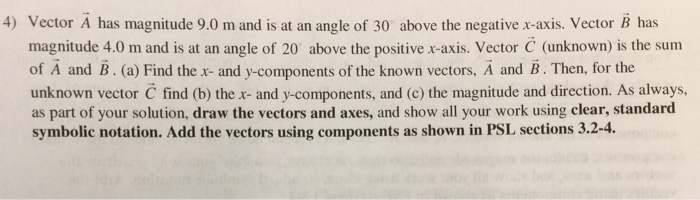Vector A has magnitude 9.0 m and is at an angle of 30 above the negative x-axis, Vector B has magnitude 4.0 m and is at an angle of 20 above the positive x-axis. Vector C (unknown) is the sum of A and B. (a) Find the x-and y-components of the known vectors, A and B. Then, for the unknown vector C find (b) the x- and y-components, and (c) the magnitude and direction. As always as part of your...

• ### 1. A hiker begins her trip away from her car by first walking 30.0 km west....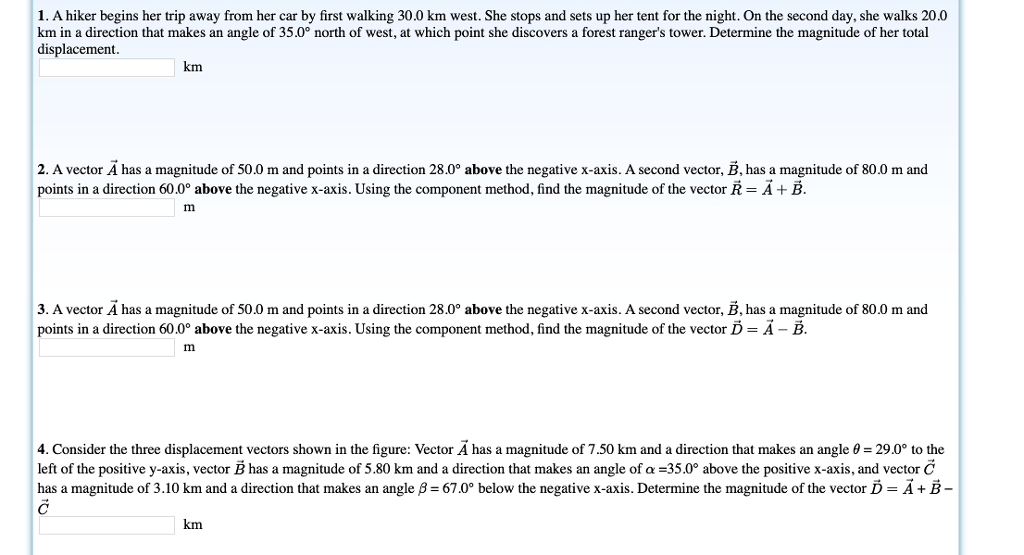1. A hiker begins her trip away from her car by first walking 30.0 km west. She stops and sets up her tent for the night. On the second day, she walks 20.0 km in a direction that makes an angle of 35.0 north of west, at which point she discovers a forest ranger's tower. Determine the magnitude of her total displacement km 2. A vector A has a magnitude of 50.0 m and points in a direction 28.0° above...

• ### physics:vectors

Two vectors and have the components, in meters, ax = -1.4, ay = 6.2, bx = 3.6, by = 1.4. (a) Find the angle between the directions of and . Round to the nearestdegree. There are two vectors in the xy plane that are perpendicular to and have a magnitude of 4.2 m. One, vector , has a positive x component and the other, vector ,a negative x component. What are (b) the x component and (c) the y component...

• ### Z- A+B+C+D Z:x component Z: y component W=A-B-C+D W :x component W: y component in the...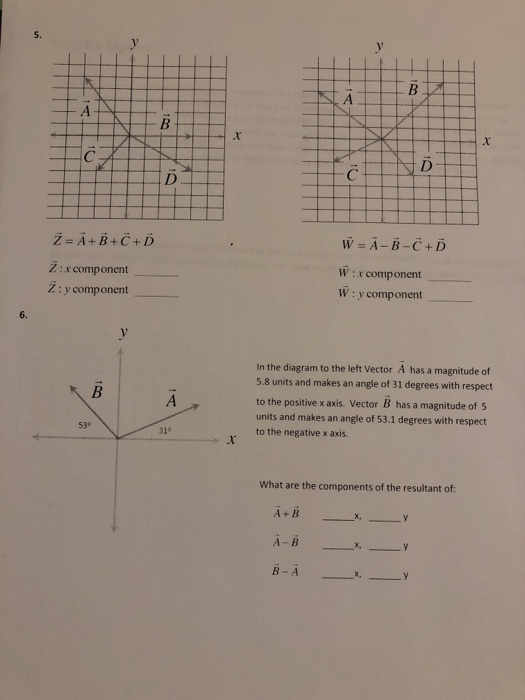Z- A+B+C+D Z:x component Z: y component W=A-B-C+D W :x component W: y component in the dagram tothe let Vecor A has a magnitude of 5.8 units and makes an angle of 31 degrees with respect to the positive x axis. Vector B has a magnitude of S units and makes an angle of 53.1 degrees with respect to the negative x axis. 53 319 What are the components of the resultant of: A+B

• ### 3. A vector A has a magnitude of 58.0 m and points in a direction 22.0°...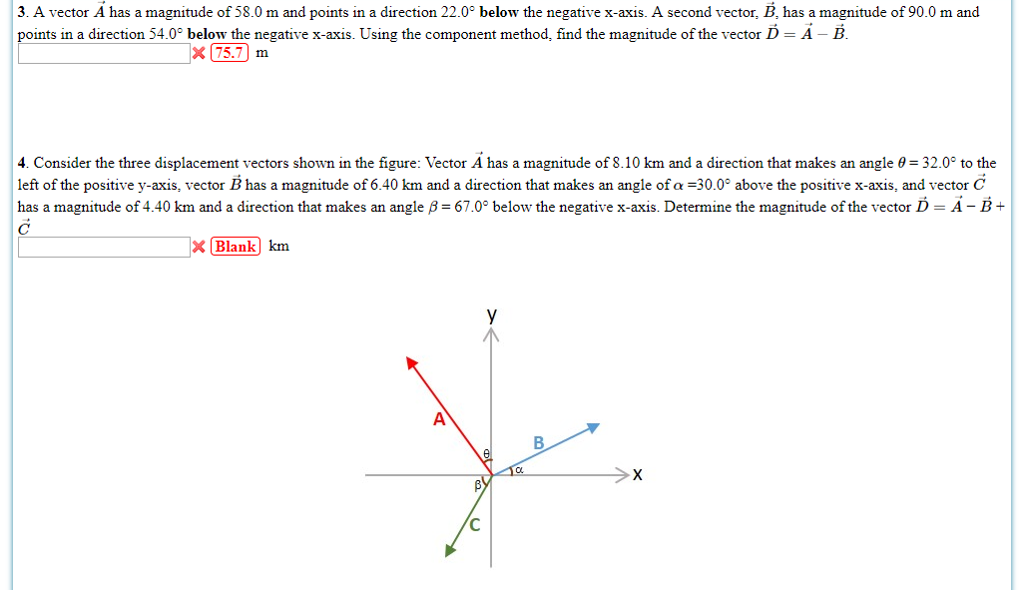3. A vector A has a magnitude of 58.0 m and points in a direction 22.0° below the negative x-axis. A second vector, B, has a magnitude of 90.0 m and points in a direction 54.0° below the negative x-axis. Using the component method, find the magnitude of the vector D - A - B x 75.7m 4. Consider the three displacement vectors shown in the figure: Vector A has a magnitude of 8.10 km and a direction that makes...

• ### 1. A force vector has a magnitude of 575 newtons and points at an angle of...

1. A force vector has a magnitude of 575 newtons and points at an angle of 36 degree below the positive x axis. What are the x scalar component and y scalar component of the vector 2. Vector A -> points along the +y axis and has a magnitude of 100.0 units. Vector B-> points at an angle of 60.0 degree above the +x axis and has a magnitude of 200.0 units. Vector C-> points along the +x axis and...

• ### Resolving Vector Components with Trigonometry 16 of 20> Part A Often a vector is specified by...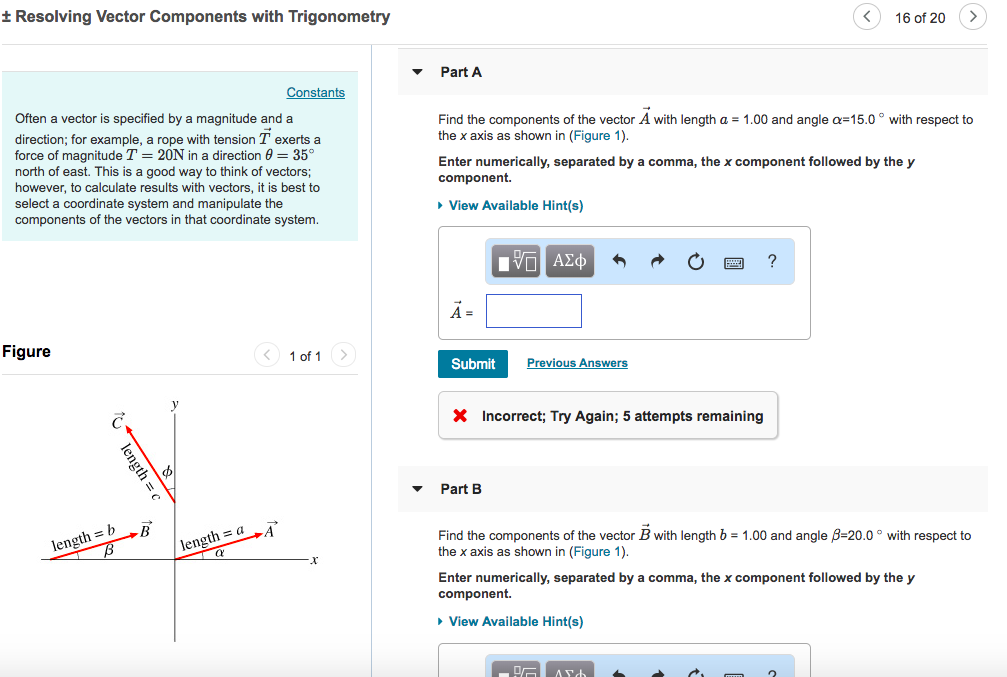Resolving Vector Components with Trigonometry 16 of 20> Part A Often a vector is specified by a magnitude and a direction; for example, a rope with tension T exerts a force of magnitude T 201 in a direction θ = 35° north of east. This is a good way to think of vectors; however, to calculate results with vectors, it is best to select a coordinate system and manipulate the components of the vectors in that coordinate system. Find the...

• ### Often a vector is specified by a magnitude and a direction; for example, a rope with tension T⃗ exerts a force of magnit...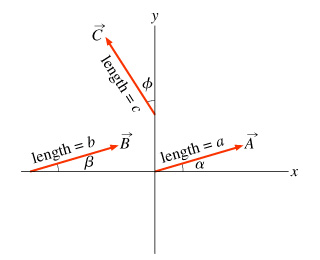Often a vector is specified by a magnitude and a direction; for example, a rope with tension T⃗ exerts a force of magnitude T=20N in a direction θ=35∘north of east. This is a good way to think of vectors; however, to calculate results with vectors, it is best to select a coordinate system and manipulate the components of the vectors in that coordinate system. Find the components of the vector B⃗ with length b = 1.00 and angle β=10.0 ∘...